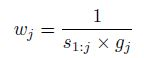## 24 mar. 2017

### Tutorials with Resemble (Part 3 - orthoProjection)

Using orthoProjection:
One of the different functions of Resemble is “orthoProjection” and we can use it with different options. Let check in this post the simplest one:
oP<-orthoProjection(Xr=der.Xr, X2 = NULL,
Yu = NULL,method = "pca",
pcSelection = list("cumvar",0.99),
center = TRUE, scaled = FALSE,
cores = 1)
We can use the training data from the previous post, with the SG filter (just for smoothing) and the first derivative: der.Xr
The method we use is “pca”, so we don´t have to use the reference data “Yr”. We don´t use any additional set so X2=NULL
The number of terms will explain a cumulative variance of 99%.
We center the spectra, and we don´t scale it.
Now run this script in R (be sure that the package Resemble is loaded, library(resemble))

Now we can check the values we get:
names(oP)
 "pcSelection" "center" "scale" "method"

>attach(oP)
>scores
Matrix T of scores
>Variance
We can see the eigenvalue, the cumulative and explained variance
>sc.sdv
eigenvalues
>n.components
Number of terms chosen to explain 99% of the variance
>pcSelection
cumvar  0,99
>center
average spectrum
>scale
1
>method
pca(svd)

Check all these values and matrices.
3.1.......Practice plotting the average spectrum. (page Exercises)
3.2.......Play with the accumulative variance.     (page Exercises)
3.4.......Plot combinations of score Maps            (page Exercises)

¡And enjoy Chemometrics with R!

## 23 mar. 2017

### Tutorials with Resemble (part 2)

If you have practise with the post : Tutorials with Resemble (part 1) , you can continue adding more script following the recomendations of the Resemble Package. This time we can add another math treatment to the previous one of the SG filter.
Once applied the "sg" function, we can calculate the first derivative to define better he variance in the spectra. The Resemble Manual show us how to convert the spectra to a first derivative using  differences. We can do it for the calibration and the validation sets:

der.Xr <- t(diff(t(Xr), lag = 1, differences = 1))
der.Xu <- t(diff(t(Xu), lag = 1, differences = 1))

In this case we lose a data point on the left of the spectra so we have to define the wavelengths to see the plot of the first derivative.

wavelength_der<-seq(1112,2488,by=2)
matplot(wavelength_der,t(der.Xr),type="l",col="black",
xlab="Wavelength(nm)",ylab="Absorbance")

and we get this plot:Practise doing the same for the validation set Xu and overplotting the spectra with the training set Xr.

Do you see significant differences?

Enjoy using Chemometrics with R.﻿
﻿

## 20 mar. 2017

### Tutorials with Resemble (part 1)

I see that some of you are interested in the package "Resemble", so I´m going to re-writte some of the post with this package, so we can understand better the LOCAL concept we have been treating with Win ISI.

The examples use the NIRsoil data that we can get from the package "prospectr".
require(prospectr)data(NIRsoil)
If we can plot the  raw spectra, ..., just writte this script
wavelength<-seq(1100,2498,by=2)
matplot(wavelength,t(NIRsoil\$spc),type="l",col="blue",
xlab="Wavelength(nm)",ylab="Absorbance",ylim=c(0,1))

In the Resemble manual recomends to apply a SG filter without derivatives to smooth the spectra, so in this case we proceed as the manual:
sg <- savitzkyGolay(NIRsoil\$spc, p = 3, w = 11, m = 0)
NIRsoil\$spc <- sg
Now the spectra is truncated in both sides, so we have to create:
wavelength_sg<-seq(1110,2488,by=2)
and we can plot the spectra filtered:
matplot(wavelength_sg,t(NIRsoil\$spc ),type="l",col="black",
xlab="Wavelength(nm)",ylab="Absorbance",ylim=c(0,1))

You won´t see too much difference with the raw spectra.

Now we split the data into a training (Xr , Yr) set and a validation set (Xu, Yu)
#VALIDATION
Xu <- NIRsoil\$spc[!as.logical(NIRsoil\$train),]
Yu <- NIRsoil\$CEC[!as.logical(NIRsoil\$train)]

#TRAINING
Xr <- NIRsoil\$spc[as.logical(NIRsoil\$train),]

Yr <- NIRsoil\$CEC[as.logical(NIRsoil\$train)]
and we take out the data without reference values form both sets:
Xu <- Xu[!is.na(Yu),]
Xr <- Xr[!is.na(Yr),]
Yu <- Yu[!is.na(Yu)]
Yr <- Yr[!is.na(Yr)]

Practise making plots again of the spectra of the diferent sets. Overlap training and validation sets with different colors,....., and enjoy using R for chemometrics.

## 6 mar. 2017

### Neighborhood Mahalanobis distance matrix

Working with the chemometric packages in R help us to understand other chemometric commercial software’s better.

In Resemble we can use the function fDiss to get a matrix of distances between all the samples in a spectra data set, so we get a square and diagonal matrix with zeroes in the diagonal, because the distance between a sample and itself in the PCA space is cero. This way we can see redundant information and remove it from the spectra set. Finally we can get a well distributed cloud of samples and the average spectrum is more representative to all of them.

Here I just trim the matrix in order to see how close the first 10 samples spectra are  between them.
The spectra used was the NIRsoil data from R.

## 5 mar. 2017

### Wheigthed Average (LOCAL)

We have seen in the post  LOCAL optimization  how, when giving a prediction, LOCAL uses all the PLS terms range we have fixed in the options Min to Max number of terms, and the result is a weighted average of all the results predictions of all the models. So to choose the right range is important to get more accurate predictions.
Looking in the Resemble R package documentation you can see some explanations about how the calculations are made:

"Weighted average pls ("wapls1"): It uses multiple models generated by multiple pls components (i.e. between a minimum and a maximum number of pls components). At each local partition the final predicted value is a weighted average of all the predicted values generated by the multiple pls models. The weight for each component is calculated as follows":"where s1:j  is the root mean square of the spectral residuals of the unknown (or target) sample when a total of j pls components are used and gj is the root mean square of the regression coefficients corresponding to the jth pls component (see Shenk et al., 1997 for more details).
"wapls1" is not compatible with valMethod = "loc_crossval" since the weights are computed based on the sample to be predicted at each local iteration.
by the multiple pls models".